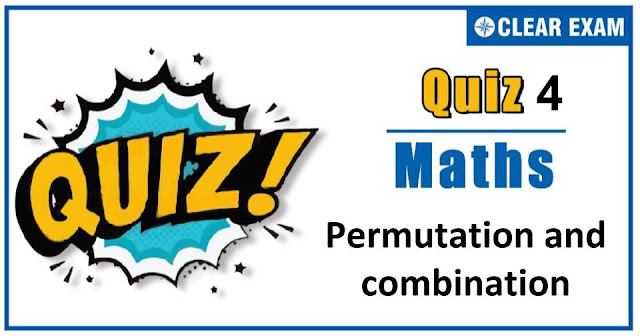## [LATEST]\$type=sticky\$show=home\$rm=0\$va=0\$count=4\$va=0

As per analysis for previous years, it has been observed that students preparing for JEE MAINS find Mathematics out of all the sections to be complex to handle and the majority of them are not able to comprehend the reason behind it. This problem arises especially because these aspirants appearing for the examination are more inclined to have a keen interest in Mathematics due to their ENGINEERING background.

Furthermore, sections such as Mathematics are dominantly based on theories, laws, numerical in comparison to a section of Engineering which is more of fact-based, Physics, and includes substantial explanations. By using the table given below, you easily and directly access to the topics and respective links of MCQs. Moreover, to make learning smooth and efficient, all the questions come with their supportive solutions to make utilization of time even more productive. Students will be covered for all their studies as the topics are available from basics to even the most advanced.

Q1. In an examination of 9 papers a candidate has to pass in more papers, then the number of papers in which he fails in order to be successful. The number of ways in which he can be unsuccessful, is
•  255
•  193
•  319
•  256
Solution
(d) ∵ The candidate is unsuccessful, if he fails in 9 or 8 or 7 or 6 or 5 papers. ∴ Numbers of ways to be unsuccessful =9C9 +9C8 +9C7 +9C6 +9C5 = 9C0+ 9C1 +9C2 +9C3 +9C4 =1/2( 9C0+9C1+.....+9C9) =1/2 (29 )=28=256

Q2.If the letters of the word KRISNA are arranged in all possible ways and these words are written out as in a dictionary, then the rank of the word KRISNA is
•  359
•  324
•  341
•  None of these
Solution
(b) The number of words starting from A are =5!=120 The number of words starting from I are =5!=120 The number of words starting from KA are =4!=24 The number of words starting from KI are =4!=24 The number of words starting from KN are =4!=24 The number of words starting from KRA are =3!=6 The number of words starting from KRIA are =2!=2 The number of words starting from KRIN are =2!=2 The number of words starting from KRISA are=1!=1 The number of words starting from KRISNA are=1!=1 Hence, rank of the word KRISNA =2(120)+3(24)+6+2(2)+2(1)=324

Q3.  There is a set of m parallel lines intersecting a set of another n parallel lines in a plane. The number of parallelograms formed, is
•   m-1C2.n-1C2
•  m-1C2.nC2
•  mC2.n-1C2
•  mC2.nC2
Solution
(d)Since, a set of m parallel lines intersecting a set of another n parallel lines in a plane, then the number of parallelograms formed is mC2.nC2.

Q4. From 12 books, the difference between number of ways a selection of 5 books when one specified book is always excluded and one specified book is always included, is
•  64
•  118
•  330
•  132
Solution
(d) Required number of ways = 11C5- 11C4 =11!/5!6!=11!/4!7!=132

Q5. The exponent of 3 in 100 !, is
•  48
•  44
•  52
•  33
Solution
(a) We have, E3 (100 !)=[100/3]+[100/32 ]+[100/33 ][100/34 ]=33+11+3+1=48

Q6. The number of all the possible selections which a student can make for answering one or more questions out of eight given questions in a paper, when each question has an alternative is
•  256
•  6560
• 6561
•  None of these
Solution
(b) Each question can be omitted or one of the two parts can be attempted i.e. it can be taken in 3 ways. So, 8 questions can be attempted in 38-1=6560 ways

Q7. The number of times the digit 5 will be written when listing the integers from 1 to 1000, is
•  271
•  272
•  300
•  None of these
Solution
(c) Since, 5 does not occur in 1000, we have to count the number of times 5 occurs when we list the integers from 1 to 999. Any number between 1 and 999 is of the form xyz,0≤x,y,z≤9 The number in which 5 occurs exactly once =(3C1 )9×9=243 The number in which 5 occurs exactly twice=(3C2.9)=27 The number in which 5 occurs in all three digits =1 Hence, the number of times 5 occurs =1×243+2×27+3×1=300

Q8. If the letters of the word LATE be permuted and the words so formed be arranged as in a dictionary. Then, the rank of LATE is
•  12
•  14
•  13
•  15
Solution
(b) 14

Q9. A student is to answer 10out of 13 questions in an examination such that he must choose at least 4 from the first five questions. The number of choices available to him is
•  140
•  280
•  346
•  196
Solution
(d) Total number of ways=5C4×8C6×5C5×8C4 =5!/(4!×1!)×8!/(2!×6!)+8!/(5!×3!) =(5×8×7)/2+(8×7×6)/6 =140+56=196

Q10. A lady gives a dinner party to 5 guests to be selected from nine friends. The number of ways of forming the party of 5, given that two of the friends will not attend the party together is
•  56
•  91
•  126
• None of these
Solution
(b) Required number = 9 C5- 7 C3=91## Want to know more

Want to Know More
Please fill in the details below:

## Latest NEET Articles\$type=three\$c=3\$author=hide\$comment=hide\$rm=hide\$date=hide\$snippet=hide

Name

ltr
item
Best IIT JEE Coaching Institute in Delhi | NEET Coaching Institute in Delhi: Permutation-and-combination-quiz-4
Permutation-and-combination-quiz-4
https://1.bp.blogspot.com/-wsuLBGivsiY/X3iv4Kp0R6I/AAAAAAAAAY8/A6ZvHiP4OwAHEfjN7tVTHUWXu2rW-4HkQCLcBGAsYHQ/w640-h336/Quiz%2BImage%2BTemplate%2B%25283%2529.jpg
https://1.bp.blogspot.com/-wsuLBGivsiY/X3iv4Kp0R6I/AAAAAAAAAY8/A6ZvHiP4OwAHEfjN7tVTHUWXu2rW-4HkQCLcBGAsYHQ/s72-w640-c-h336/Quiz%2BImage%2BTemplate%2B%25283%2529.jpg
Best IIT JEE Coaching Institute in Delhi | NEET Coaching Institute in Delhi
https://www.cleariitmedical.com/2020/10/permutation-and-combination-quiz-4.html
https://www.cleariitmedical.com/
https://www.cleariitmedical.com/
https://www.cleariitmedical.com/2020/10/permutation-and-combination-quiz-4.html
true
7783647550433378923
UTF-8